Courses

# Crystals MCQ Level – 1

## 10 Questions MCQ Test Topic wise Tests for IIT JAM Physics | Crystals MCQ Level – 1

Description
This mock test of Crystals MCQ Level – 1 for Physics helps you for every Physics entrance exam. This contains 10 Multiple Choice Questions for Physics Crystals MCQ Level – 1 (mcq) to study with solutions a complete question bank. The solved questions answers in this Crystals MCQ Level – 1 quiz give you a good mix of easy questions and tough questions. Physics students definitely take this Crystals MCQ Level – 1 exercise for a better result in the exam. You can find other Crystals MCQ Level – 1 extra questions, long questions & short questions for Physics on EduRev as well by searching above.
QUESTION: 1

### Which of the following has bcc structure?

Solution: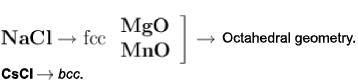QUESTION: 2

### If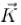is wave vector of incident X-ray and be the reciprocal lattice vector then condition for Bragg reflection is given by :

Solution:

Let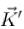be the scattered wave vector, then from Ewald construction :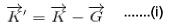Since, for elastic scattering, there will be no change in the magnitude of the vector i.e.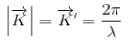Now squaring (i),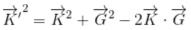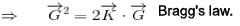The correct answer is: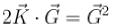QUESTION: 3

### Which of the following lattices has the least packing fraction?

Solution:

packing fraction of simple cubic = 52% packing fraction of bee = 68%
packing fraction of fee = 74%
packing fraction of diamond = 34%

QUESTION: 4

The radii of Cs and Cl are r1 and r2 respectively. Which of the following gives the lattice constant of CsCI if it is crystalized in bcc structure :

Solution:

For bcc structure ,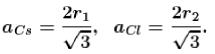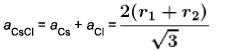The correct answer is: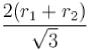QUESTION: 5

A lattice is characterized by following primitive vectors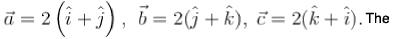Solution: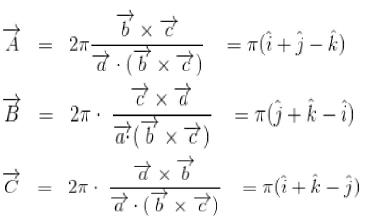Hence, lattice is bcc with edge π
The correct answer is: bcc with cube edge π

QUESTION: 6

What is the reciprocal lattice to simple cubic lattice?

Solution:

Primitive translation Vectors of simple cubic lattice may be written as :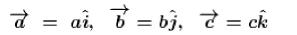Then primitive translation vector of reciprocal lattice is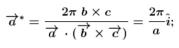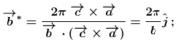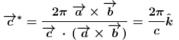Hence, the reciprocal lattice is itself a simple cubic lattice with lattice constant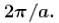The correct answer is: simple cubic

QUESTION: 7

A body centred cubic lattice has edge a. Edge of its primitive cells will be :

Solution: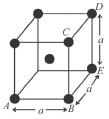from figure, AB = a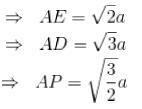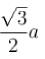QUESTION: 8

The primitive translation vectors of the fcc lattice are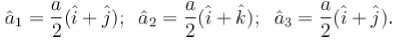The volume of the primitive cell of reciprocal lattice is :

Solution:

The primitive translation vector of the reciprocal lattice to the above lattice be calculated as :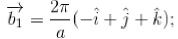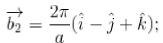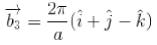Volume of the reciprocal lattice is :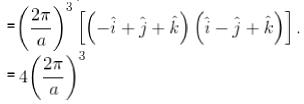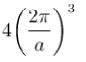QUESTION: 9

The positions of number of nearest neighbours in a unit cell of bcc structure is given by:

Solution: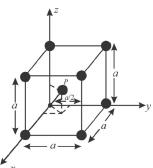Nearest neighbour to an atom (say at origin) is the atom present is the centre of the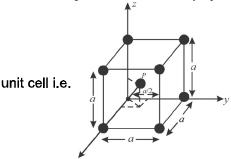at point P and the position of P can be =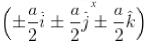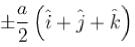QUESTION: 10

What is the second nearest neighbour distance in a face centered cubic whose conventional unit cell parameter is a?

Solution: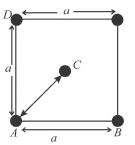Here AC is the nearest distance while AB or AD forms the nearest neighbour distance which is equal to ' a '.